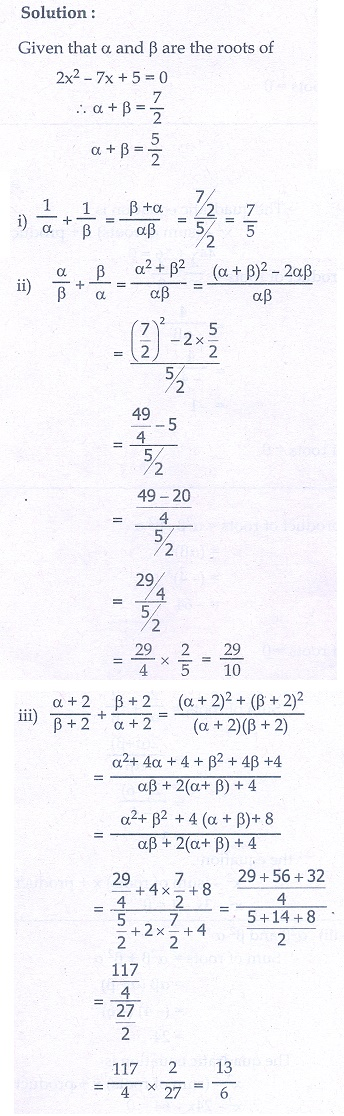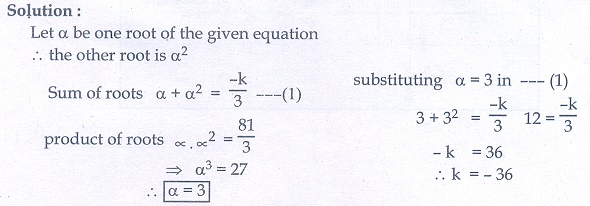Home | | Maths 10th Std | Exercise 3.14: The Relation between Roots and Coefficients of a Quadratic Equation

# Exercise 3.14: The Relation between Roots and Coefficients of a Quadratic Equation

Maths Book back answers and solution for Exercise questions - Mathematics : Algebra: The Relation between Roots and Coefficients of a Quadratic Equation: Exercise Problem Questions with Answer

### Exercise 3.14

1. Write each of the following expression in terms of α + β and αβ .(iii) (3α - 1)(3β -1)2. The roots of the equation 2x2 − 7x + 5 = 0 are α and β. Without solving for the roots, find3. The roots of the equation x2 + 6x − 4 = 0 are α, β. Find the quadratic equation whose roots are

(i) α 2  and β 2

(ii) 2/α and 2/β

(iii) α2β and β2α4. If α, β are the roots of 7x2 + ax + 2 = 0 and if β − α =  -13/7. Find the values of a.5. If one root of the equation 2y2 − ay + 64 = 0 is twice the other then find the values of a.6. If one root of the equation 3x2 + kx + 81 = 0 (having real roots) is the square of the other then find k.